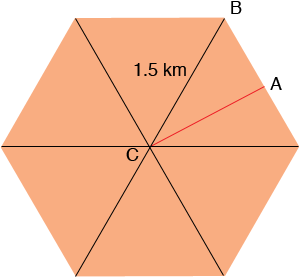Quandaries and Queries Name: bhavin Who is asking: Student Level of the question: All Question: i have a radius of hexagon given as 1.5 km. and i want to find the area of that hexagon. Hi Bhavin, I expect that you mean a regular hexagon. Join each vertex of the hexagon to the centre C of the hexagon to form 6, congruent triangles. The six angles at the center of the hexagon are of equal measure so each measures  360/6 = 60 degrees.In the diagram above, A is the midpoint of a side and hence the measure of angle CAB is 90 degrees, the measure of angle BCA is 30 degrees and the measure of angle ABC is 60 degrees. This should allow you to find the lengths of CA and AB and hence the area of triangle CAB. The area of the hexagon is 12 times the area of the triangle CAB. Penny